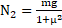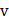# A sphere is placed rotating with its centre initially at rest in a corner as shown in figures (a) and (b). Coefficient of friction between all surfaces and the sphere is 1/3. Find the ratio of the friction forcesby ground in situation (a) and (b)a) 1 b)c)d) None of these

## Question ID - 100233 :- A sphere is placed rotating with its centre initially at rest in a corner as shown in figures (a) and (b). Coefficient of friction between all surfaces and the sphere is 1/3. Find the ratio of the friction forcesby ground in situation (a) and (b)a) 1 b)c)d) None of these

3537

(b)

In figure, for translational equilibrium in vertical directionIn horizontal direction…(ii)

Solving.Eqs. (i) and (ii),The required friction isIn figure, the sphere has the tendency to move toward the right;Which givesNext Question :
 A particle of masstravelling with velocityand kinetic energycollides elastically to another particle of mass, at rest. What is the fraction of total energy retained by the particle of mass? a)b)c)d) None of these